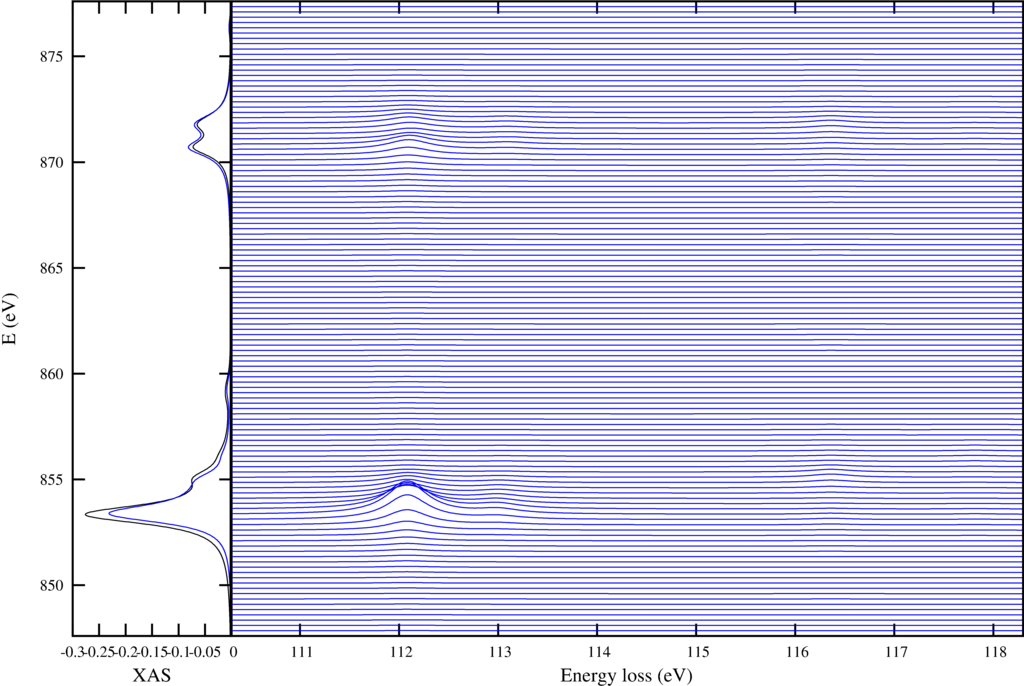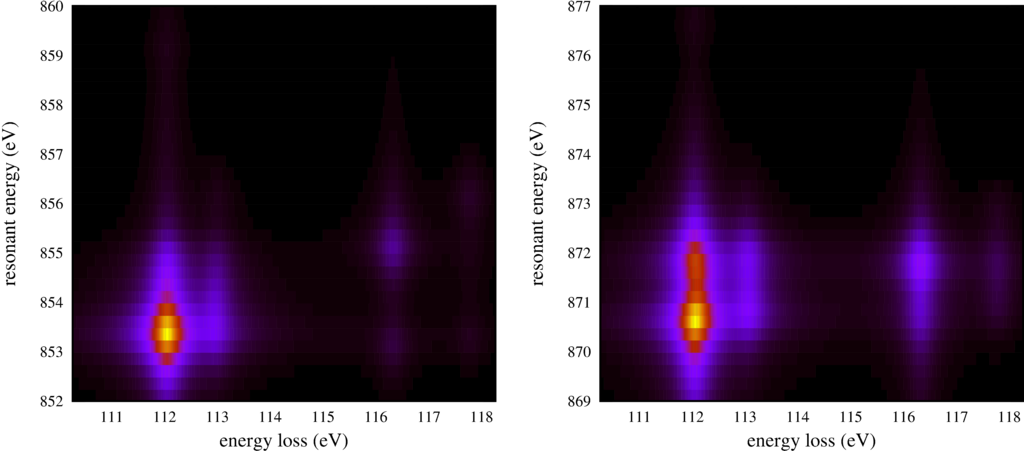# RIXS $L_{2,3}M_{1}$

Instead of looking at low energy excitations one can use RIXS to look at core-core excitations. A powerful technique comparable to other core level spectroscopies like x-ray absorption and core-level photoemission at once.

Here a script to calculate the Ni $2p$ to $3d$ excitation ($L_{2,3}$) and Ni $3s$ to $2p$ decay ($M_{1}$).

RIXS_L23M1.Quanty
-- This example calculates core - core RIXS spectra. These type of spectra are
-- highly informative and contain similar information as core level absorption and
-- core level photoemission combined. With generally enhances sensitivity.

-- we focus here on 2p to 3d excitations (L23) and 3s to 2p decay (final hole in the 3s
-- state, the M1 edge)

-- We use the definitions of all operators and basis orbitals as defined in the file
-- include and can afterwards directly continue by creating the Hamiltonian
-- and calculating the spectra

dofile("Include.Quanty")

-- The parameters and scheme needed is the same as the one used for XAS

-- We follow the energy definitions as introduced in the group of G.A. Sawatzky (Groningen)
-- J. Zaanen, G.A. Sawatzky, and J.W. Allen PRL 55, 418 (1985)
-- for parameters of specific materials see
-- A.E. Bockquet et al. PRB 55, 1161 (1996)
-- After some initial discussion the energies U and Delta refer to the center of a configuration
-- The L^10 d^n   configuration has an energy 0
-- The L^9  d^n+1 configuration has an energy Delta
-- The L^8  d^n+2 configuration has an energy 2*Delta+Udd
--
-- If we relate this to the onsite energy of the L and d orbitals we find
-- 10 eL +  n    ed + n(n-1)     U/2 == 0
--  9 eL + (n+1) ed + (n+1)n     U/2 == Delta
--  8 eL + (n+2) ed + (n+1)(n+2) U/2 == 2*Delta+U
-- 3 equations with 2 unknowns, but with interdependence yield:
-- ed = (10*Delta-nd*(19+nd)*U/2)/(10+nd)
-- eL = nd*((1+nd)*Udd/2-Delta)/(10+nd)
--
-- For the final state we/they defined
-- The 2p^5 L^10 d^n+1 configuration has an energy 0
-- The 2p^5 L^9  d^n+2 configuration has an energy Delta + Udd - Upd
-- The 2p^5 L^8  d^n+3 configuration has an energy 2*Delta + 3*Udd - 2*Upd
--
-- If we relate this to the onsite energy of the p and d orbitals we find
-- 6 ep + 10 eL +  n    ed + n(n-1)     Udd/2 + 6 n     Upd == 0
-- 6 ep +  9 eL + (n+1) ed + (n+1)n     Udd/2 + 6 (n+1) Upd == Delta
-- 6 ep +  8 eL + (n+2) ed + (n+1)(n+2) Udd/2 + 6 (n+2) Upd == 2*Delta+Udd
-- 5 ep + 10 eL + (n+1) ed + (n+1)(n)   Udd/2 + 5 (n+1) Upd == 0
-- 5 ep +  9 eL + (n+2) ed + (n+2)(n+1) Udd/2 + 5 (n+2) Upd == Delta+Udd-Upd
-- 5 ep +  8 eL + (n+3) ed + (n+3)(n+2) Udd/2 + 5 (n+3) Upd == 2*Delta+3*Udd-2*Upd
-- 6 equations with 3 unknowns, but with interdependence yield:
-- epfinal = (10*Delta + (1+nd)*(nd*Udd/2-(10+nd)*Upd) / (16+nd)
-- edfinal = (10*Delta - nd*(31+nd)*Udd/2-90*Upd) / (16+nd)
-- eLfinal = ((1+nd)*(nd*Udd/2+6*Upd)-(6+nd)*Delta) / (16+nd)
--
-- note that ed-ep = Delta - nd * U and not Delta
-- note furthermore that ep and ed here are defined for the onsite energy if the system had
-- locally nd electrons in the d-shell. In DFT or Hartree Fock the d occupation is in the end not
-- nd and thus the onsite energy of the Kohn-Sham orbitals is not equal to ep and ed in model
-- calculations.
--
-- note furthermore that ep and eL actually should be different for most systems. We happily ignore this fact
--
-- We normally take U and Delta as experimentally determined parameters
--
-- besides the two configurations with either no core hole or the configuration with one
-- core hole in the 2p shell we now also need a configuration with one core hole in the
-- 3s shell
--
-- We define:
-- The 3s^1 L^10 d^n+1 configuration has an energy 0
-- The 3s^1 L^9  d^n+2 configuration has an energy   Delta +   Udd -   Usd
-- The 3s^1 L^8  d^n+3 configuration has an energy 2*Delta + 3*Udd - 2*Usd
--
-- If we relate this to the onsite energy of the s and d orbitals we find
-- 2 es + 10 eL +  n    ed + n(n-1)     Udd/2 + 2 n     Usd == 0
-- 2 es +  9 eL + (n+1) ed + (n+1)n     Udd/2 + 2 (n+1) Usd == Delta
-- 2 es +  8 eL + (n+2) ed + (n+1)(n+2) Udd/2 + 2 (n+2) Usd == 2*Delta+Udd
-- 1 es + 10 eL + (n+1) ed + (n+1)(n)   Udd/2 + 1 (n+1) Usd == 0
-- 1 es +  9 eL + (n+2) ed + (n+2)(n+1) Udd/2 + 1 (n+2) Usd == Delta+Udd-Usd
-- 1 es +  8 eL + (n+3) ed + (n+3)(n+2) Udd/2 + 1 (n+3) Usd == 2*Delta+3*Udd-2*Usd
--
-- 6 equations with 3 unknowns, but with interdependence yield:
-- eswiths = (10*Delta + (1+nd)*(nd*Udd/2-(10+nd)*Usd) / (12+nd)
-- edwiths = (10*Delta - nd*(23+nd)*Udd/2-22*Usd) / (12+nd)
-- eLwiths = ((1+nd)*(nd*Udd/2+2*Usd)-(2+nd)*Delta) / (12+nd)

-- number of electrons (formal valence)
nd = 8
-- parameters from experiment (core level PES)
Udd     =  7.3
Upd     =  8.5
Usd     =  6.0
Delta   =  4.7
-- parameters obtained from DFT (PRB 85, 165113 (2012))
F2dd    = 11.14
F4dd    =  6.87
F2pd    =  6.67
G1pd    =  4.92
G3pd    =  2.80
G2sd    = 12.56
tenDq   =  0.56
tenDqL  =  1.44
Veg     =  2.06
Vt2g    =  1.21
zeta_3d =  0.081
zeta_2p = 11.51
Bz      =  0.000001
H112    =  0.120

ed      = (10*Delta-nd*(19+nd)*Udd/2)/(10+nd)
eL      = nd*((1+nd)*Udd/2-Delta)/(10+nd)

epfinal = (10*Delta + (1+nd)*(nd*Udd/2-(10+nd)*Upd)) / (16+nd)
edfinal = (10*Delta - nd*(31+nd)*Udd/2-90*Upd) / (16+nd)
eLfinal = ((1+nd)*(nd*Udd/2+6*Upd) - (6+nd)*Delta) / (16+nd)

eswiths = (10*Delta + (1+nd)*(nd*Udd/2-(10+nd)*Usd)) / (12+nd)
edwiths = (10*Delta - nd*(23+nd)*Udd/2-22*Usd) / (12+nd)
eLwiths = ((1+nd)*(nd*Udd/2+2*Usd) - (2+nd)*Delta) / (12+nd)

F0dd    = Udd + (F2dd+F4dd) * 2/63
F0pd    = Upd + (1/15)*G1pd + (3/70)*G3pd
F0sd    = Usd + G2sd/10

Hamiltonian    =  F0dd*OppF0_3d + F2dd*OppF2_3d + F4dd*OppF4_3d + zeta_3d*Oppldots_3d + Bz*(2*OppSz_3d + OppLz_3d) + H112 * (OppSx_3d+OppSy_3d+2*OppSz_3d)/sqrt(6) + tenDq*OpptenDq_3d + tenDqL*OpptenDq_Ld + Veg * OppVeg + Vt2g * OppVt2g + ed      * OppN_3d + eL      * OppN_Ld

XASHamiltonian =  F0dd*OppF0_3d + F2dd*OppF2_3d + F4dd*OppF4_3d + zeta_3d*Oppldots_3d + Bz*(2*OppSz_3d + OppLz_3d) + H112 * (OppSx_3d+OppSy_3d+2*OppSz_3d)/sqrt(6) + tenDq*OpptenDq_3d + tenDqL*OpptenDq_Ld + Veg * OppVeg + Vt2g * OppVt2g + edfinal * OppN_3d + eLfinal * OppN_Ld + epfinal * OppN_2p + zeta_2p * Oppcldots + F0pd * OppUpdF0 + F2pd * OppUpdF2 + G1pd * OppUpdG1 + G3pd * OppUpdG3

Hamiltonian3s  =  F0dd*OppF0_3d + F2dd*OppF2_3d + F4dd*OppF4_3d + zeta_3d*Oppldots_3d + Bz*(2*OppSz_3d + OppLz_3d) + H112 * (OppSx_3d+OppSy_3d+2*OppSz_3d)/sqrt(6) + tenDq*OpptenDq_3d + tenDqL*OpptenDq_Ld + Veg * OppVeg + Vt2g * OppVt2g + edwiths * OppN_3d + eLwiths * OppN_Ld + eswiths * OppN_3s                       + F0sd * OppUsdF0 + G2sd * OppUsdG2

-- we now can create the lowest Npsi eigenstates:
Npsi=3
-- in order to make sure we have a filling of 8 electrons we need to define some restrictions
StartRestrictions = {NF, NB, {"000000 00 1111111111 0000000000",8,8}, {"111111 11 0000000000 1111111111",18,18}}

psiList = Eigensystem(Hamiltonian, StartRestrictions, Npsi)
oppList={Hamiltonian, OppSsqr, OppLsqr, OppJsqr, OppSx_3d, OppLx_3d, OppSy_3d, OppLy_3d, OppSz_3d, OppLz_3d, Oppldots_3d, OppF2_3d, OppF4_3d, OppNeg_3d, OppNt2g_3d, OppNeg_Ld, OppNt2g_Ld, OppN_3d}

-- print of some expectation values
print("  #    <E>      <S^2>    <L^2>    <J^2>    <S_x^3d> <L_x^3d> <S_y^3d> <L_y^3d> <S_z^3d> <L_z^3d> <l.s>    <F>   <F>   <Neg^3d> <Nt2g^3d><Neg^Ld> <Nt2g^Ld><N^3d>");
for i = 1,#psiList do
io.write(string.format("%3i ",i))
for j = 1,#oppList do
expectationvalue = Chop(psiList[i]*oppList[j]*psiList[i])
io.write(string.format("%8.3f ",expectationvalue))
end
io.write("\n")
end

-- spectra XAS
XASSpectra = CreateSpectra(XASHamiltonian, T2p3dx, psiList, {{"Emin",-10}, {"Emax",20}, {"NE",3500}, {"Gamma",1.0}})
XASSpectra.Print({{"file","RIXSL23M1_XAS.dat"}})

-- spectra FY
FYSpectra = CreateFluorescenceYield(XASHamiltonian, T2p3dx, T3s2py, psiList, {{"Emin",-10}, {"Emax",20}, {"NE",3500}, {"Gamma",1.0}})
FYSpectra.Print({{"file","RIXSL23M1_FY.dat"}})

-- spectra RIXS
RIXSSpectra = CreateResonantSpectra(XASHamiltonian, Hamiltonian3s, T2p3dx, T3s2py, psiList, {{"Emin1",-10}, {"Emax1",20}, {"NE1",120}, {"Gamma1",1.0}, {"Emin2",-1}, {"Emax2",9}, {"NE2",1000}, {"Gamma2",0.5}})
RIXSSpectra.Print({{"file","RIXSL23M1.dat"}})

print("Finished calculating the spectra now start plotting.\nThis might take more time than the calculation");

-- and make some plots
gnuplotInput = [[
set autoscale
set xtic auto
set ytic auto
set style line  1 lt 1 lw 1 lc rgb "#000000"
set style line  2 lt 1 lw 1 lc rgb "#FF0000"
set style line  3 lt 1 lw 1 lc rgb "#00FF00"
set style line  4 lt 1 lw 1 lc rgb "#0000FF"

set out 'RIXSL23M1_Map.ps'
set terminal postscript portrait enhanced color  "Times" 8 size 7.5,6

unset colorbox

energyshift=857.6
energyshiftM1=110.8

set multiplot
set size 0.5,0.55
set origin 0,0

set ylabel "resonant energy (eV)" font "Times,10"
set xlabel "energy loss (eV)" font "Times,10"

set yrange [852:860]
set xrange [energyshiftM1-0.5:energyshiftM1+7.5]

plot "<(awk '((NR>5)&&(NR<1007)){for(i=3;i<=NF;i=i+2){printf \"%s \", $i}{printf \"%s\", RS}}' RIXSL23M1.dat)" matrix using ($2/100-1.0+energyshiftM1):($1/4+energyshift-10):(-$3) with image notitle

set origin 0.5,0

set yrange [869:877]
set xrange [energyshiftM1-0.5:energyshiftM1+7.5]

plot "<(awk '((NR>5)&&(NR<1007)){for(i=3;i<=NF;i=i+2){printf \"%s \", $i}{printf \"%s\", RS}}' RIXSL23M1.dat)" matrix using ($2/100-1.0+energyshiftM1):($1/4+energyshift-10):(-$3) with image notitle

unset multiplot

set out 'RIXSL23M1_Spec.ps'
set size 1.0, 1.0
set terminal postscript portrait enhanced color  "Times" 8 size 7.5,5

set multiplot
set size 0.25,1.0
set origin 0,0

set ylabel "E (eV)" font "Times,10"
set xlabel "XAS" font "Times,10"
set yrange [energyshift-10:energyshift+20]
set xrange [-0.3:0]
plot "RIXSL23M1_XAS.dat"  using       3:($1+energyshift) notitle with lines ls 1,\ "RIXSL23M1_FY.dat" using (-5*$2):($1+energyshift) notitle with lines ls 4 set size 0.8,1.0 set origin 0.2,0.0 set xlabel "Energy loss (eV)" font "Times,10" unset ylabel unset ytics set xrange [energyshiftM1-0.5:energyshiftM1+7.5] ofset = 0.25 scale=10 plot for [i=0:120] "RIXSL23M1.dat" using ($1+energyshiftM1):(-column(3+2*i)*scale+ofset*i-10 + energyshift) notitle with lines ls  4

unset multiplot
]]

-- write the gnuplot script to a file
file = io.open("RIXSL23M1.gnuplot", "w")
file:write(gnuplotInput)
file:close()

-- call gnuplot to execute the script
os.execute("gnuplot RIXSL23M1.gnuplot")
-- transform to pdf and eps
os.execute("ps2pdf RIXSL23M1_Map.ps  ; ps2eps RIXSL23M1_Map.ps  ;  mv RIXSL23M1_Map.eps temp.eps  ; eps2eps temp.eps RIXSL23M1_Map.eps  ; rm temp.eps")
os.execute("ps2pdf RIXSL23M1_Spec.ps ; ps2eps RIXSL23M1_Spec.ps ;  mv RIXSL23M1_Spec.eps temp.eps ; eps2eps temp.eps RIXSL23M1_Spec.eps ; rm temp.eps")

Just like in the case of $L_{2,3}M_{4,5}$ edge RIXS we can make plots:

 Resonant inelastic x-ray scattering spectra for different incoming and outgoing photon energies.The first shows on the left the XAS spectra in black and the integrated RIXS spectra (FY) in blue. The core-core RIXS is shown on the right.

 Resonant inelastic x-ray scattering spectra for different incoming and outgoing photon energies.The second plot shows the same RIXS spectra, but now as an intensity map.

The output of the script is:

RIXS_L23M1.out
  #    <E>      <S^2>    <L^2>    <J^2>    <S_x^3d> <L_x^3d> <S_y^3d> <L_y^3d> <S_z^3d> <L_z^3d> <l.s>    <F>   <F>   <Neg^3d> <Nt2g^3d><Neg^Ld> <Nt2g^Ld><N^3d>
1   -3.503    1.999   12.000   15.095   -0.370   -0.115   -0.370   -0.115   -0.741   -0.230   -0.305   -1.042   -0.924    2.186    5.990    3.825    6.000    8.175
2   -3.395    1.999   12.000   15.160   -0.002   -0.000   -0.002   -0.000   -0.003   -0.001   -0.322   -1.043   -0.925    2.189    5.988    3.823    6.000    8.178
3   -3.286    1.999   12.000   15.211    0.369    0.113    0.369    0.113    0.737    0.227   -0.336   -1.043   -0.925    2.193    5.987    3.820    6.000    8.180
Start of LanczosTriDiagonalizeKrylovMC
Start of LanczosTriDiagonalizeKrylovMC
Finished calculating the spectra now start plotting.
This might take more time than the calculation

Workshops

Script versionsExternal programsPrivacy

##### Tools